PSAT Math : Expressions

Example Questions

1 2 3 4 6 Next →

Example Question #1 : How To Add Rational Expressions With A Common Denominator

Simplify the following rational expression: (9x - 2)/(x2) MINUS (6x - 8)/(x2)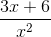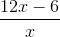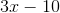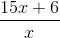Explanation:

Since both expressions have a common denominator, x2, we can just recopy the denominator and focus on the numerators. We get (9x - 2) - (6x - 8). We must distribute the negative sign over the 6x - 8 expression which gives us 9x - 2 - 6x + 8 ( -2 minus a -8 gives a +6 since a negative and negative make a positive). The numerator is therefore 3x + 6.

Example Question #1 : Rational Expressions

Simplify the expression.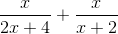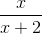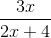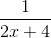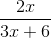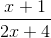Explanation:

To add rational expressions, first find the least common denominator. Because the denominator of the first fraction factors to 2(x+2), it is clear that this is the common denominator. Therefore, multiply the numerator and denominator of the second fraction by 2.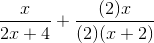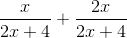This is the most simplified version of the rational expression.

Example Question #1 : How To Evaluate Rational Expressions

If √(ab) = 8, and a= b, what is a?

10

16

4

64

2

4

Explanation:

If we plug in a2 for b in the radical expression, we get √(a3) = 8. This can be rewritten as a3/2 = 8. Thus, loga 8 = 3/2. Plugging in the answer choices gives 4 as the correct answer.

Example Question #4 : Rational Expressions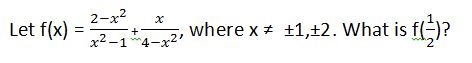–11/5

–37/15

9/5

37/15

–9/5

–11/5

Explanation: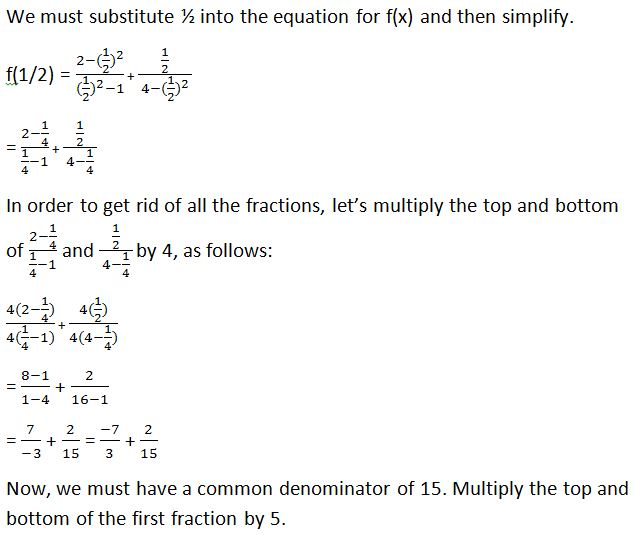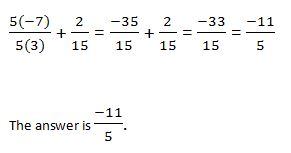Example Question #1 : How To Subtract Rational Expressions With Different Denominators

Simplify.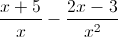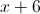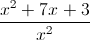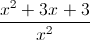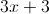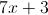Explanation:Determine an LCD (Least Common Denominator) betweenand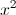.

LCD =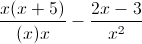Multiply the top and bottom of the first rational expression by, so that the denominator will be.

Distribute theto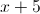.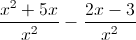Now you can subtract because both rational expressions have the same denominators.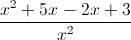Example Question #1 : Rational Expressions

Which of the following is equivalent to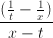? Assume that denominators are always nonzero.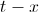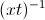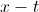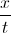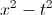Explanation:

We will need to simplify the expression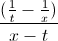. We can think of this as a large fraction with a numerator of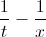and a denominator of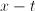.

In order to simplify the numerator, we will need to combine the two fractions. When adding or subtracting fractions, we must have a common denominator.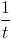has a denominator of, and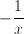has a denominator of. The least common denominator that these two fractions have in common is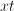. Thus, we are going to write equivalent fractions with denominators of.

In order to convert the fraction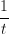to a denominator with, we will need to multiply the top and bottom by.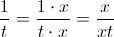Similarly, we will multiply the top and bottom ofby.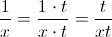We can now rewriteas follows:=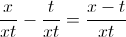Let's go back to the original fraction. We will now rewrite the numerator:=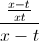To simplify this further, we can think ofas the same as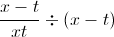. When we divide a fraction by another quantity, this is the same as multiplying the fraction by the reciprocal of that quantity. In other words,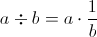.=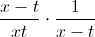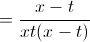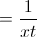Lastly, we will use the property of exponents which states that, in general,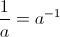.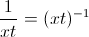The answer is.

Example Question #1 : Rational Expressions

Simplify (4x)/(x– 4) * (x + 2)/(x– 2x)

x/(x + 2)

4/(x + 2)2

4/(x – 2)2

(4x+ 8x)/(x+ 8x)

x/(x – 2)2

4/(x – 2)2

Explanation:

Factor first.  The numerators will not factor, but the first denominator factors to (x – 2)(x + 2) and the second denomintaor factors to x(x – 2).  Multiplying fractions does not require common denominators, so now look for common factors to divide out.  There is a factor of x and a factor of (x + 2) that both divide out, leaving 4 in the numerator and two factors of (x – 2) in the denominator.

Example Question #9 : Rational Expressions

what is 6/8 X 20/3

18/160
5
9/40
120/11
3/20